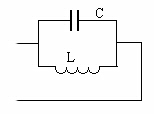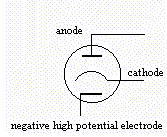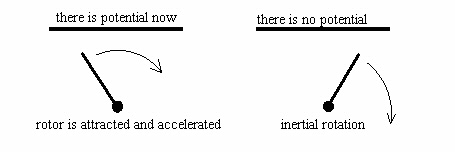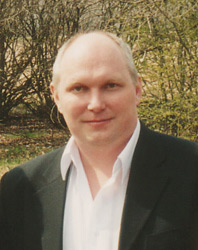Power from Nothing
Inner structure of zero
Alexander V. Frolov
P.O.Box 37, 193024, St.-Petersburg, Russia
alex@frolov.spb.ru
Introduction

The vacuum in some sense is "empty  place"  or  "nothing" but the question for extraction of energy from vacuum  is  widely discussed. This article is description of approach  that  can  be
developed in technologies to create power in load, the  Work,  in other words, by means of equal and intercompensating processes.

Premises

Simple mathematical formulation

1 + (-1) = 0               F.1

can be reversed in next view

0 = 1 + (-1)               F.2

that  show  logical  possibility  to  create  two  values    from "nothing". Note that term "zero" is not  "absolute  nothing"  but "zero" have inner structure. Zero can be created as sum  for  two opposite values, negative and positive. Also zero can  be  created as result of interaction for three values and for many values:

A + B + C = 0              F.4

0 = A + B + C              F.5

For many-polarity is possible to create several values from "nothing".

Let's appeal to definition of Power. The Power is

P = A/T                    F.6

where P is power, A is work,  T is time of work

So, the power is description  of  Process  of certain time period. And the Work for transmission of some mass by definition is

A = F S                    F.7

where F is some force that produce this work, S is the distance of transmission

Now let's try find possibility for creation of power from nothing. By F.2 this possibility is

0 = P + (-P)               F.8

It means that it is impossible to create something  from  nothing but it is possible to create two opposite  power  processes  from nothing. It is way for structuring (or ordering) of zero-state.

The way to produce P and -P is defined by two factors:  from  F.6 it is factor of time "t"

0 = P(t) + P(-t)           F.9

and from F.7 it is factor of space "S"

0 = P(S) + P(-S)           F.10

Experimental Examples

In any electromagnetics textbook one can find   the description of LC resonance circuit. For parallel LC circuit it is Fig.1 schemeFigure 1

This well-known device demonstrate possibility to  create  in  C-part of circuit current with +(T/4) phase outstripping and in  L-part of circuit the current have -(T/4) phase delay. So, currents in  C-part  and  in  L-part  have 180 degrees phase displacement. From the point of view of source that is  connected to A-B there are no any current in this case. If  amperemeter  in C wire and amperemeter in L wire  are  demonstrating  the  same value, the amperemeter in input common wire will show zero-current.
The resonance is wonderful phenomenon that allow to create "t"  and  "-t"  factors  to  use  two  opposite processes without any power consumption from source.

Now let us consider formulation F.10. Any potential field is real demonstration of this formulation  since  total  work  of potential field is sum for  positive  work  (  acceleration)  and
negative work (deceleration). All we  need  to  create  power  by means of "zero-process"  like  potential  field  is  division  of positive and negative work of field. Simple example  for  divided
trajectory is special vacuum tube where factor "S" is used.Figure 2

The electrical circuit  have  part  from  cathode  to  anode  and potential field created by screen  make  additional  acceleration for emission current electrons. Note that in ordinary vacuum tube
the screen is placed between cathode and  anode  to  control  the flow of emission electrons. Theoretically, current in anode  wire must be more powerful than current in cathode wire.

So, it is possible to use  potential field only for  acceleration of charged particles to produce useful  work in load. Electrical potential field can be used in pulsed mode to  produce acceleration of rotor. Electrostatical interaction is  well-known but mainly as some statical mode. There are difference in principle for pulsed potential and for pulsed electrical current. First  is sufficient to produce power free but second  require the current and power from the primary source. Example is Fig.3Figure 3

Segment of rotor is attracted to plate when the plate have electrical potential and acceleration have  place.  When  rotor  had  passed vertical position for this scheme, the deceleration will be made, so the plate should be disconnected from potential source. The device must use the electric induction or displacement currents but not conductivity current.

So, factor "t" from F.9 also can be used for division of work  of electrical potential field to create power from it.

Conclusion

In  general  sense  the  same  method  can  be  used   in conception for  matter  and  reverse-time  matter  (anti-matter). Universe, atom, electron and so on can exist by this way  without
any power consumption.

If a free energy technologies are using "time-factor"  by F.9 and "space-factor" by F.10, some  chronal  and  gravitational  effects in area of  these free energy process can be detected. It
is  so call "three-sided effect": energy, time and gravity are in three-lateral connection.

Term "information" is available for  free  energetics.  For the case above, Fig.1 there are two equal processes are existing in strength of the source that is the source for changing  potential  only.  It  is  "source  of information" for "power-creating circuit". But it is not  "source of power". The same situation was considered by N.Tesla. He wrote that radio receiver take power from either, not from transmitter. The  consumption  power  of transmitter  is  not  increasing when many receivers are in operating mode.

Example for informational exchange is  phenomenon  of  electrical induction.  There is the magnet analogy for it also: the magnetization or magnet induction.

Free energetics is energy-information technology.  Power  can  be considered as result for change of informational state, or result of change of form of energy. For zero-state, for "nothing", for vacuum there is no some certain defined form of energy The Process that is created in free energy generator determine the  type  and quantity of energy. When structure  of "zero" is defined by  some way  (two  opposite  processes, for example) the power, the mass, the space and the time of this energy process are created.